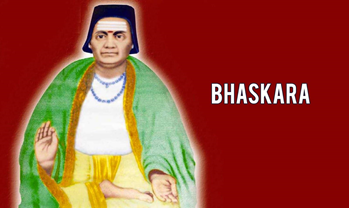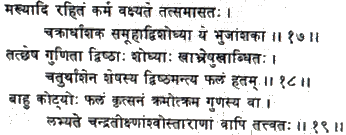Mathematics
Bhāskara i - Great Indian Mathematician
15.2k views

 1 Introduction 2 Who is Bhaskara i? 3 Works of Bhaskara i 4 Summary 5 FAQs

28 September 2020

## IntroductionBhāskara i (c. 600 – c. 680) was a 7th-century Indian mathematician and astronomer. He is referred to as Bhaskara i in order to differentiate from the 12th-century mathematician Bhaskara. Bhaskara-i is considered to be one of the three pearls of Indian Astronomy and Mathematics along with Brahmagupta and Madhava Samgramagrama.

## Who is Bhāskara i?

Bhāskaracharya was a famous mathematician but not much is known about his early life except what has been inferred from his writings. Many believe that he must have been working in a school of mathematicians in Asmaka which was probably in the Nizamabad District of Andhra Pradesh. There are other references to places in India in Bhaskara's writings. There are some allusions to Valabhi (today Vala), the capital of the Maitraka dynasty in the 7th century, and Sivarajapura, which were both in Saurashtra, which today is the state of Gujarat. There is yet another school of thought which believes that he was born in Bori, in Parbhani district of Maharashtra. By and large, it is believed that Bhaskara was born in Saurashtra and later moved to Asmaka. He was tutored in astronomy by his father.

It is believed his father taught him astronomy. Bhaskara i is considered to be a follower of Aryabhata. He is considered to be the most important scholar of Aryabhata's astronomical school.

Bhaskara i is famous for the following works:

1. Zero, positional arithmetic, the approximation of sine.

2. The three treatises he wrote on the works of Aryabhata (476–550 CE )

• The Aryabhatiyabhashya (629)

### Zero, positional arithmetic, approximation of sine

One of the most important mathematical contributions is related to the representation of numbers in a positional system. The first positional representations were known to Indian astronomers about 500 years ago before Bhaskaracharya, but the numbers were not written in figures, but in words, symbols or pictorial representations. For example, the number 1 was given as the moon, since there is only one moon. The number 2 was represented anything in pairs; the number 5 could relate to the five senses and so on…

He explains a number given in this system, using the formula ankair api, ("in figures, this reads") by repeating it written with the first nine Brahmi numerals, using a small circle for the zero. Brahmi numerals system, dating from 3rd century B.C is an ancient system for writing numerals and are the direct graphic ancestors of the modern Indian and Hindu-Arabic numerals. Since 629, the decimal system has been known to the Indian scientists. Though Bhaskara i did not invent it, he was the first to use the Brahmi numerals in a scientific contribution in Sanskrit.

### Bhaskara I's sine approximation formula

Bhaskara i knew the approximation to the sine functions that yields close to 99% accuracy, using a function that is simply a ratio of two quadratic functions.

The formula is given in verses 17 – 19, Chapter VII, Mahabhaskariya of Bhaskara I. He stated the formula in stylised verse.

According to his formula:

If 0 $$\le \text{ x } ≤ 180$$ then sin x deg is approximately equal to $$\frac{4x(180-x)}{(40500-x(180-x))}$$

\begin{align}&\text{Sin a}=\frac{4a(180-a)}{40500-a(180-a)}\end{align}Below is briefly stated the rule for finding the bhujaphala  ( result from the base sine)and the kotiphala, etc.) The result obtained by multiplying the R sine of the koṭi due to the planet's kendra by the tabulated epicycle and dividing the product by 80 without making use of the Rsine-differences 225, etc.

Subtract the degrees of a bhuja (or koti) from the degrees of a half-circle (that is, 180 degrees). Then multiply the remainder by the degrees of the bhuja or koti and put down the result at two places. At one place subtract the result from 40500. By one-fourth of the remainder (thus obtained), divide the result at the other place as multiplied by the 'anthyaphala (that is, the epicyclic radius). Thus is obtained the entire bahuphala (or, kotiphala) for the sun, moon or the star-planets. So also are obtained the direct and inverse Rsines.

It is not known how Bhaskara I arrived at his approximation formula though many historians of mathematics have marveled at the accuracy of the formula. The formula is simple and enables one to compute reasonably accurate values of trigonometric sines without using any geometry whatsoever.

The Mahabhaskariya is a work on Indian mathematical astronomy consisting of eight chapters dealing with mathematical astronomy. The book deals with topics such as the longitudes of the planets; association of the planets with each other, conjunctions among the plant and the stars; the lunar crescent; solar and lunar eclipses; and rising and setting of the planets. As referred to earlier, this treatise also includes chapters which illustrate the sine approximation formula. Both the treatises, Mahabhaskariya knew and Laghubhaskariya''), are astronomical works in verse. It is interesting to note that Parts of Mahabhaskariya were later translated into Arabic.

### The Aryabhatiyabhashya (629)

The Aryabhatiyabhashya is Bhaskara I’s commentary on the Aryabhatiya. The Aryabhatiya is a treatise on astronomy written in Sanskrit. It is said to be the only known surviving work of the 5th-century Indian mathematician Aryabhata. It is estimated that the book was written around 510 B.C.

Bhaskara I wrote the Aryabhatiyabhasya in 629

Bhaskara I’s comments revolve around the 33 verses in Aryabhatiya which is about mathematical astronomy. He also expounds on the problems of indeterminate equations and trigonometric formulas. While discussing Aryabhatiya, he discussed cyclic quadrilaterals. He was the first mathematician to discuss quadrilaterals whose four sides are not equal with none of the opposite sides parallel. Bhaskara i explains in detail Aryabhata’s method of solving linear equations with illustrative examples.

He stressed on the need for providing mathematical rules.

## Summary:

It would not be wrong to say that the Bhaskara i has played a pivotal role in the lasting influence of Aryabhata’s works. Befitting a mathematician of his stature, the Indian Space Research Organisation launched Bhaskara I honouring the mathematician on 7 June 1979.

Mathematicians have agreed that Bhaskara i’s sine approximation formula is fairly accurate for all practical purposes. The formula, whether in its original form or modified versions has been used by authors down the line. Keeping in mind its origins centuries ago, it reflects a very high standard of mathematics in India at that time. Truly wondrous to imagine how the seeds of modern science were sown centuries ago!